1. Worksheets>
2. Math>
3. Number Sense>
4. Odd and Even Numbers

# Odd and Even Numbers Worksheets

Numerous odd and even number worksheets have printable exercises to color the objects in odd and even places, to group the objects, to count them and classify them as odd or even, to complete odd and even series and cutting and pasting activities. Mazes and chart pdfs are also available here. These odd and even numbers worksheets are suitable for children in grade 1 through grade 4. Try out some of these worksheets for free!

Odd / Even Charts

Amusing charts for children to study the odd and even numbers are given under different themes. Print and paste these charts in your study room.Charts: Odd / Even numbers

Coloring odd / even number of objects

Each worksheet has seven different sets of objects. Color the objects in the odd place or the even place.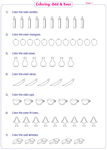Grouping the items

In these worksheets, circle the colorful objects into two groups. The entire group can be an odd group or an even group or a combination of both groups.Coloring Activity: Under the sea

An interesting under sea theme is given with a variety of fishes. Color the odd bubbles blue and even bubbles green in each grade 1 worksheet.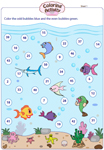Count and identify odd / even

Objects of everyday use are shown in groups. Count the objects and write down the total. Classify the total as odd or even.Group in pairs

Circle the objects into groups of two in these printable worksheets for 1st grade and 2nd grade kids. Count the objects and classify them as odd or even.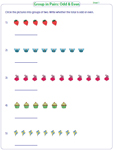Write odd or even: Themed

Each pdf worksheet carries a unique sport theme. Race cars, race horses and sports t-shirts are displayed with different numbers. Write whether odd or even.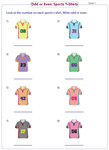Write odd or even: Standard

These worksheets contain 18 questions (2-digit or 3-digit numbers). Write if the numbers are odd or even in the space provided.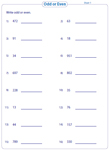Identifying odd / even numbers

Circle the odd and even numbers from the list of numbers. Two MCQs are also included in each worksheet.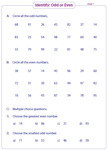Sum / Difference

In these printable worksheets for grade 2 and grade 3, find whether the sum or difference is odd or even without actually performing the addition / subtraction operation.Complete the pattern

Attractive animals for 3rd grade and 4th grade children are shown in these pdf worksheets. They carry either an odd series or an even series. Fill in the missing numbers.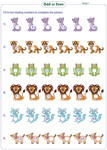Forming odd / even numbers

In section A, write the smallest and the greatest odd or even numbers formed by the given digits. In section B, write all the possible odd / even numbers formed by the given digits.Odd / Even Maze

Find the path from the entrance to the goal. Enjoy this maze activity by circling or coloring the odd and even numbers.Maze: Odd / Even numbers

Related Worksheets